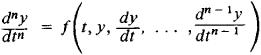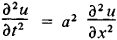# Initial Condition

Also found in: Acronyms.

## initial condition

[i′nish·əl kən′dish·ən]
(computer science)
(meteorology)
A prescription of the state of a dynamical system at some specified time; for all subsequent times the equations of motion and boundary conditions determine the state of the system; the appropriate synoptic weather charts, for example, constitute a (discrete) set of initial conditions for a forecast; in many contexts, initial conditions are considered as boundary conditions in the dimension of time.

## Initial Condition

in the mathematical analysis of a process the state of the process at a given moment of time, taken as the initial moment. If the process is described by a differential equation, then the problem of finding solutions for a given initial condition is called the Cauchy problem. An initial condition for the equationconsists in specifying y, dy/dt, . . . , (dn-1y)/dtn-1 for a value t = t0. If n = 2 and y = y(t) is the law of motion of a point mass, then the initial condition specifies the position and velocity of the point at the moment t = t0 An initial condition for a partial differential equation is similarly defined. Thus, for the equationof a free vibrating string, where u(t, x) is the deviation of the point x of the string at the moment t from the position of equilibrium on the x-axis, the initial condition specifies the initial shape uǀt = t0 = f(x) of the string and the initial velocities δu/ δ/1,= t0 of the points of the string. Any other argument may play the role of time. An initial condition is then specified for some value of that argument.

References in periodicals archive ?
Despite the consistent behaviors of the real-time intensity error growth with those inferred from the HSD and RE models, we should point out that the above-mentioned real-time error growth analysis contains some inherent uncertainties due to several factors, such as a limited sample size, a lack of detailed analyses of the model vortex's initial condition, inadequate representation of hurricane physics, and even discrepancies between the model pointwise maximum surface wind and the observed intensity.
We obtain the initial condition for the chaotic logistic map by substituting [y.
The task of finding of periodic solutions of nonlinear differential equations is more complex than the Cauchy task of integrating of these equations from the initial conditions to establishment because it imposes another condition on the solution--the condition of periodicity, that is it becomes a point-to-point T-periodic boundary value problem of nonlinear differential equations.
Finally, the claim of the hyperchaotic system for the chosen parameters and the initial condition by authors in  could not be established through our numerical simulations.
Weremark that the initial condition (41) is oscillatory, and Schrodinger equation (3) propagates oscillations of wave length O(s) in space and timeWe choose an appropriately long interval [a, b] for the computations such that the zero far-field boundaryconditions (5) do not introduce a significant error relative to the whole space problemAs already pointed out, the main goal when solvingSchrodinger equation is to compute macroscopic quantities (e.
The specification of the initial conditions will require the potential-energy surface (PES) of the XH[Y.
To study the global stability of the limit-cycle solutions obtained by solving equation (2), we investigate the motion of the MEMS capacitor for a set of initial conditions in the proximity of either the stable or unstable fixed points.
Three factors constituted this part of the experiment: wood species, initial condition of the raw material (lumber) for primary size reduction (shredding), and particle size obtained from primary size reduction.
A trajectory of a DI over I with the initial condition [x.
Lozanovski is against the demand for destroying this object and returning the place to its initial condition.
0](t) denotes an initial guess of the exact solution Y(t) which satisfies the initial condition (7).

Site: Follow: Share:
Open / Close